Courses

# Metal Oxide Semiconductor Field Effect Transistor (MOSFET) Capacitor (Part - 2) Electrical Engineering (EE) Notes | EduRev

## VLSI System Design

Created by: Machine Experts

## Electrical Engineering (EE) : Metal Oxide Semiconductor Field Effect Transistor (MOSFET) Capacitor (Part - 2) Electrical Engineering (EE) Notes | EduRev

The document Metal Oxide Semiconductor Field Effect Transistor (MOSFET) Capacitor (Part - 2) Electrical Engineering (EE) Notes | EduRev is a part of the Electrical Engineering (EE) Course VLSI System Design.
All you need of Electrical Engineering (EE) at this link: Electrical Engineering (EE)

Objectives

In this course you will learn the following

• Threshold Voltage Calculation
• C-V characteristics
• Oxide Charge Correction

5.1 Threshold Voltage Calculation

Threshold voltage is that gate voltage at which the surface band bending is twice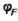,Where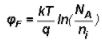We know that the depth of depletion region for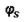is between 0 and 2and is given by,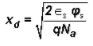Charge in depletion region at= 2is given by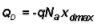where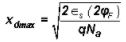Beyond threshold, the total charge QD in the seminconductor has to balance the charge on gate electrode, Qs i.e.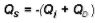where we define the charge in the inversion layer as a quantity which needs to be determined.

This leads to following expression for gate voltage-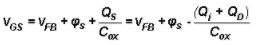In case of depletion, there in no inversion layer charge, so Qi =0, i.e. gate voltage becomes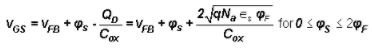but in case of inversion, the gate voltage will be given by :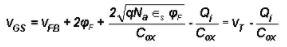The second term in second equality of last expression states our basic assumption, namely that any change in gate voltage beyond the threshold requires a change in inversion layer charge. Also from the same expression, we obtain threshold voltage as :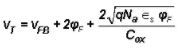5.2 C-V Characteristics

The low frequency and high frequency C-V characteristics curves of a MOS capacitor are shown in fig 5.2.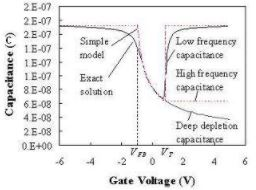Fig 5.2 : Low & High Frequency C-V curves

The low frequency or quasi-static measurement maintains thermal equilibrium at all times. This capacitance is the ratio of the change in charge to the change in gate voltage, measured while the capacitor is in equilibrium. A typical measurement is performed with an electrometer, which measures the charge added per unit time as one slowly varies the applied gate voltage.

The high frequency capacitance is obtained from a small-signal capacitance measurement at high frequency. The bias voltage on the gate is varied slowly to obtain the capacitance versus voltage. Under such conditions, one finds that the charge in the inversion layer does not change from the equilibrium value corresponding to the applied DC voltage. The high frequency capacitance therefore reflects only the charge variation in the depletion layer and the (rather small) movement of the inversion layer charge.

5.3 Oxide Charge Correction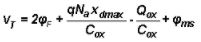To keep the value of Vr within -1 Volt and +1 Volt, an n-channel device has high Na doping (similarly, p-channel device has high Nd doping).

Offer running on EduRev: Apply code STAYHOME200 to get INR 200 off on our premium plan EduRev Infinity!

,

,

,

,

,

,

,

,

,

,

,

,

,

,

,

,

,

,

,

,

,

;